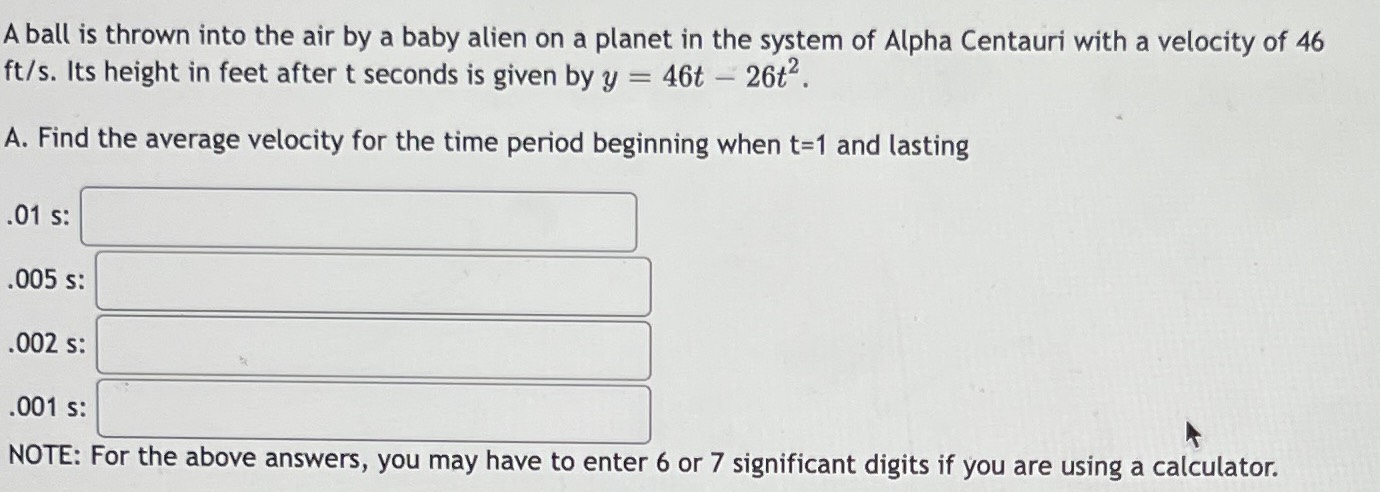### ¿Todavía tienes preguntas de matemáticas?

Pregunte a nuestros tutores expertos
Algebra
PreguntaA ball is thrown into the air by a baby alien on a planet in the system of Alpha Centauri with a velocity of $$46$$ $$ft / s$$ . Its height in feet after $$t$$ seconds is given by $$y = 46 t - 26 t ^ { 2 }$$ .

A. Find the average velocity for the time period beginning when $$t = 1$$ and lasting

NOTE: For the above answers, you may have to enter $$6$$ or $$7$$ significant digits if you are using a calculator.